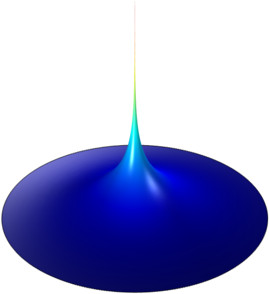# Application Gallery

#### Implementing a Point Source

Application ID: 73

This model solves the Poisson equation on a unit disk with a point source in the origin. The easiest way to describe a point source in COMSOL Multiphysics is by using an extra weak term.

To obtain the weak formulation of the general Poisson equation, we multiply it with a test function u_test and integrate over the domain. The mesh density is dense, close to the origin, so as to resolve the singularity. The solution is compared to the analytical solution.This model example illustrates applications of this type that would nominally be built using the following products: